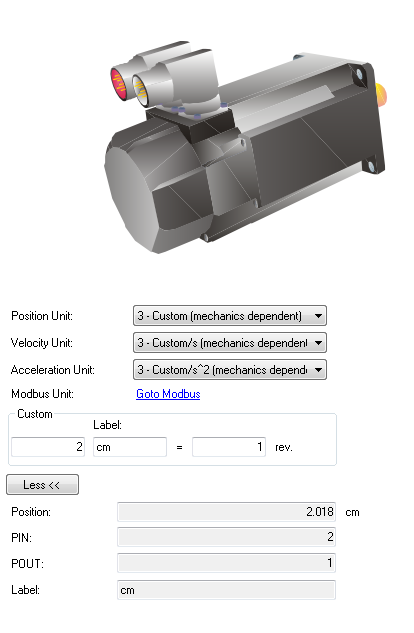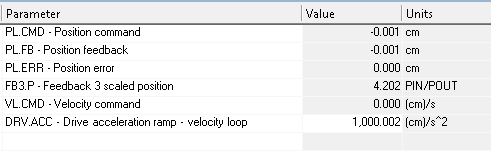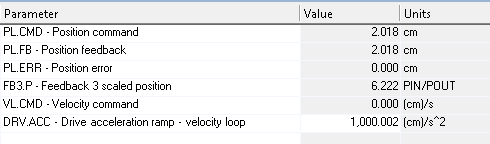EnDat 2.2 encoder set as a tertiary feedback.

Firmware version: M-01-12-01-000

This article explains how to set an EnDat 2.2 encoder as tertiary feedback (FB3) in AKD. The article contains:

Introduction

Note: The configuration of EnDat 2.2 as tertiary feedback is only supported with drives: AKD-x-xxxxx-NBxx-xxxx

AKD offers the possibility to connect a second feedback for position reference. If the second feedback is an EnDat 2.2 encoder, then the whole configuration is actually called tertiary feedback. More information of how to connect an EnDat 2.2 as tertiary feedback can be found in the “AKD user Guide” or under following link:

Kollmorgen WorkBench Help - Encoder Emulation

Initial settings

Following parameters should be set to recognize an EnDat 2.2 as tertiary feedback.

 DRV.EMUEMODE = 11 FB3 Input as tertiary feedback PL.FBSOURCE = 2 Tertiary feedback connected to X9

Parameters

The following table shows which FB3 parameters should be also configured:

Examples

Note: The following examples do not include a gear box.

1. Commissioning a rotary motor with a SFD as primary feedback and a rotary feedback EnDat 2.2 as tertiary feedback with a resolution of 1 rev = 232 counts.

1. Read a position from FB3. As previous step, be sure that your EnDat 2.2 encoder is sending a position value. Therefore, set DRV.EMUEMODE=11 and PL.FBSOURCE=0 and check that AKD is reading a position. If the position is being read, a value in FB3.P should be shown.
2. Set the units for FB3. Through FB3.PUNIT you can set the units for FB3.P as counts or customer units (FB3.PIN / FB3.POUT). In this example, we select FB3.PUNIT = 0, then FB3.P = counts
3. Set the resolution of FB3. Now the resolution of your tertiary feedback should be set in FB3.ENCRES. As default FB3.ENCRES = 0 and means a resolution of 1 rev = 232 counts. In this example, our FB3 has a resolution of 232, therefore FB3.ENCRES = 0. To ensure that your resolution is correct and is set correctly, move your shaft one revolution and FB3.P should increase in a step of 232.
4. Set EnDat 2.2 as tertiary feedback. For this, you should set PL.FBSOURCE = 2. After that PL.FB is the same or directly proportional to FB3.P, depending on UNIT.PROTARY, UNIT.PIN and UNIT.POUT.
5. Customize counting direction and sign of FB3. So far FB3 is giving a correct position to PL.FB. There are still three variables to customize FB3.P according to your application. Two of them (FB3.DIR and FB3.PDIR) are responsible for the counting direction and sign of FB3.P. In the following, every combination of FB3.DIR and FB3.PDIR and the values of FB3.P are shown. Such values are based on an initial position, while the shaft is rotated 90 degrees in positive and negative (Positive means: having the motor shaft in front of you, it is rotated clockwise).

If FB3.DIR = 0 AND FB3.PDIR = 0

• Initial position + 90° -> PL.FB = FB3.P = 4 197 152 555 912
• Initial position = 0°   -> PL.FB = FB3.P = 4 196 095 204 864
• Initial position -90°   -> PL.FB = FB3.P = 4 195 012 530 800

If FB3.DIR = 1 AND FB3.PDIR = 0

• Initial position + 90° -> PL.FB = FB3.P = 4 195 277 413 840
• Initial position = 0°   -> PL.FB = FB3.P = 4 196 207 245 336
• Initial position -90°   -> PL.FB = FB3.P = 4 197 332 750 920

If FB3.DIR = 0 AND FB3.PDIR = 1

• Initial position + 90° -> PL.FB = -FB3.P = -4 197 152 555 912
• Initial position = 0°   -> PL.FB = -FB3.P = -4 196 095 204 864
• Initial position -90°   -> PL.FB = -FB3.P = -4 195 012 530 800

If FB3.DIR = 1 AND FB3.PDIR = 1

• Initial position + 90° -> PL.FB = -FB3.P = -4 195 277 413 840
• Initial position = 0°   -> PL.FB = -FB3.P = -4 196 207 245 336
• Initial position -90°   -> PL.FB = -FB3.P = -4 197 332 750 920
1. Customize an offset. If you should include an offset, set it through FB3.POFFSET. The value of such offset is based on FB3.PUNIT. In this example, FB3.POFFSET = 1 000 000 000

If FB3.POFFSET = 0,                      then FB3.P = 4 196 207 245 336

If FB3.POFFSET = 1 000 000 000, then FB3.P = 4 197 207 245 336

1. Considering the same motor of example I, but this time the (rotary) tertiary feedback device is place at the load which has a 1:4 gear ratio (motor does four revolutions per revolution of tertiary feedback) and the customer has a gear IN of 360 and a gear out of 1. Besides, the position should be in customer units
1. Read a position from FB3. Here the procedure is the same.
2. Set the units for FB3. The customer application has a gear IN of 360 and a gear OUT of 1, therefore, the following parameters should be set: FB3.PIN = 360, FB3.POUT = 1. To define the customer units, set FB3.PUNIT = 3. So far, 1 rev = 360PIN/POUT.
3. Set the resolution of FB3. Although the position is in customer units, the resolution FB3.ENCRES is always in counts. For the example, the load has a gear ratio 1:4, then FB3.ENCRES = 232/gear ratio 232/4

Steps 4, 5 and 6 are the same as example I.

The examples I and II are based on the same rotary motor with the same (rotary) tertiary feedback for PL.FB, but in example I the gear ratio (load: motor) is 1:1 while in example II is 1:4. Now, lets commission an linear EnDat 2.2 encoder

1. Commissioning a rotary motor with a SFD as primary feedback and a linear feedback EnDat 2.2 as tertiary feedback, with a resolution of 5nm and the relationship between rotary motor and linear feedback is 2cm = 1rev.
1. Get ready units. Before you start commissioning the tertiary feedback, set the factors and units in such way that makes the understanding and analysis easier. As we know, motor and tertiary feedback have a factor 2cm = 1rev. Set this custom factor as follows: UNIT.PIN = 2, UNIT.LABEL = cm, UNIT.POUT = 1rev. Now set the units as custom units as follows: UNIT.PROTARY = UNIT.VROTARY = UNIT.ACCROTARY = 3 that represent cm, cm/s and cm/s2 respectively (see Fig. 1).
2. Read a position from FB3. Here the procedure is the same.
3. Set the units for FB3. The customer application has a relationship IN of 2cm and a gear OUT of 1rev, therefore, the following parameters should be set: FB3.PIN = 2, FB3.POUT = 1. To define the customer units, set FB3.PUNIT = 3. So far, 1 rev = 2PIN/POUT.
4. Set the resolution of FB3. Although the position is in customer units, the resolution FB3.ENCRES is always in counts. For the example, we have a relationship 2cm = 1rev and the encoder resolution is 5nm, then FB3.ENCRES = 2cm/5nm = 4000000.

Steps 4, 5 and 6 are the same as example I.Figure 1: UNIT

The following figures show how your variables and units look at one initial position and after 2 cm

InitialFigure 2: Initial variables

After moving 2cmFigure 3: variables after moving 2cm

AttachmentEnDat22 as tertiary feedback - KDN Article.pdf